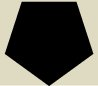# Discrete Geometry II

## Summer Semester 2015

newstimessyllabusliteraturehomeworkswhat happened so far

### news

All updates will posted on the mailing list. Please make sure you subscribed!
• the wiki is on
• new lecture times!
• class starts Tuesday, April 14

### times

 lecture tuesday 10-12 Arnimallee 6 SR 007/008 wednesday 14-16 Arnimallee 6 SR 007/008recitation thursday 10-12 Arnimallee 3 SR 119### syllabus / prerequisites / formalities

This is the second in a series of three courses on discrete geometry. The aim of the course is a skillful handling of discrete geometric structures with an emphasis on convex geometric properties. In the course we will develop central themes in discrete and convex geometry including proof techniques and applications to other areas in mathematics. The material will be a selection of the following topics:

Basic structures in discrete and convex geometry

• convexity and separation theorems
• convex bodies and polytopes/polyhedra
• faces and boundary structures
• polarity
• approximation by polytopes
• subdivisions and triangulations (including Delaunay and Voronoii)
Notions and tools
• face numbers and invariants
• volumes and mixed volumes
• volume computations and estimates
• Löwner-John ellipsoids and roundness
• valuations
Geometric inequalities
• face numbers and invariants
• Brunn-Minkowski and Alexsandrov-Fenchel inequality
• isoperimetric inequalities
• measure concentration and phenomena in high-dimensions
• Minkowski's representation theorem for polytopes
• face numbers and invariants
Geometry of numbers
• lattices
• Minkowski's (first) theorem
• successive minima
• lattice points in convex bodies and Ehrhart's theorem
• Ehrhart-Macdonald reciprocity
Sphere packings
• lattice packings and coverings
• Theorem of Minkowski-Hlawka
• analytic methods
The target audience are students with an interest in discrete mathematics and (convex) geometry. The course is a good entry point for a specialization in discrete geometry. The topics addressed in the course supplement and deepen the understanding for discrete-geometric structures appearing in differential geometry, topology, combinatorics, and algebraic geometry.

#### prerequisites

Solid background in linear algebra and some analysis. Basic knowledge and experience with polytopes and/or

#### Formalities

To successfully pass the course, you need to...
• ...actively participate in the recitations,
• ...get at least 60 percent of the total number of homework points (see homeworks),
• ...present at least one of the homework problems in the recitation, and
• ...pass the exam at the end of the semester (details will be discussed in the first week).

### literature

A good part of the mentioned books is in the Semesterapparat at the math library.

### homeworks

There will be one homework sheet per week (posted here). You have to hand them in Wednesday before the lecture. More information will come soon. Hand in the solutions in pairs. Let us know if you couldn't find a partner. Try to solve all the problems but mark two solutions. Only these will be graded. (The supplementary problems do not count; they are for your enjoyment.) You can earn 20 points on every homework sheet (10 per exercise). There are marked problems that are mandatory. You can get extra credit by solving the bonus problems. State who wrote up the solution. Everybody has to write up at least 25 percent of the solutions. Everybody has to present at least one problem in the recitation session. Everybody has to write down the solution of one problem in the wiki, it will be graded as an additional homework sheet. You can get extra credit by adding and editing entries of the wiki.
You can add/edit solutions for some homework exercises in the wiki: http://www.mathseminar.org/DiscreteGeometryII.
 Homework #1 due April 22 Homework #2 due April 29 Homework #3 due May 6 Homework #4 due May 13 Homework #5 due May 20 Homework #6 due May 27 Homework #7 due June 3 Homework #8 due June 10 Homework #9 due June 24 Homework #10 due July 7 Bonus Homework due July 21### what happened so far

You can find LaTeXed lecture notes here. Disclaimer: they are not necessarily complete, they are not necessarily correct. Don't rely on these notes - take your own! If you find errors or short-comings, please let me know.

 May 12/13 flags of faces; barycentric subdivision; pyramids and volumes; general formula for volume; Minkowski's theorem and the easy half; beneath-beyond and placing triangulations -- a practical algorithm May 5/6 distance function of a convex body; membership/separation oracles; outer parallel bodies and Hausdorff distance; Relation to support functions; approximations of convex bodies by polytopes; Volume of convex bodies I: Volume as Jordan measure; polyboxes and Jordan measure; Volume from scratch: dissections and Hilbert's 3rd problem; Volume of a simplex and extensions to all polytopes; April 28/29 polarity; properties; Minkowski-Weyl for polytopes; faces, facets; conjugate faces finitely generated and polyhedral cones; polars of cones; Farkas lemma; digression: linear programming, weak and strong duality; support functions; Theorem: support functions are exactly the sublinear (positively homogeneous and subadditive) functions; the convex cone of convex bodies April 21/22 extreme points and Minkowski's theorem: A convex body is the convex hull of its extreme points; nearest point maps characterize closed convex sets; separating hyperplanes and a separation theorem; supporting hyperplanes and the support function; the monoid of convex bodies; proof of Minkowski's theorem; faces and exposed faces; face lattice; faces vs. exposed faces; faces of polytopes are exposed April 14/15 5 minutes to fall in love with discrete convex geometry; linear/affine subspaces, linear/affine hulls; segments and convex sets; Examples: unit balls and norms, convex cone of positive semi-definite matrices Examples (cont'd): positive polynomials, sums-of-squares, epigraphs and convex functions; convex hulls and polytopes; convex combinations; Caratheodory's theorem; (relative) interior and boundary; affine hull and dimension; convex hull of compact set is compact